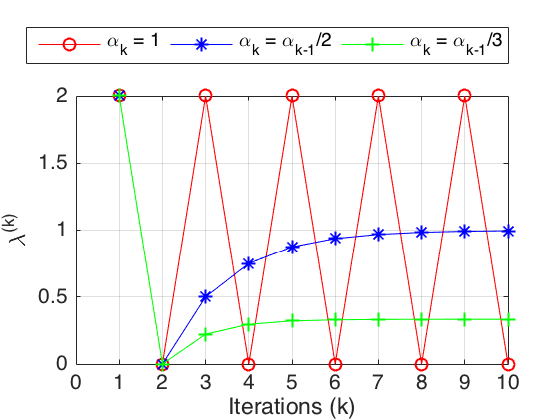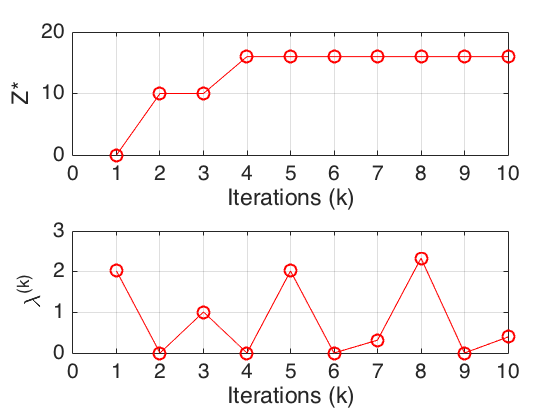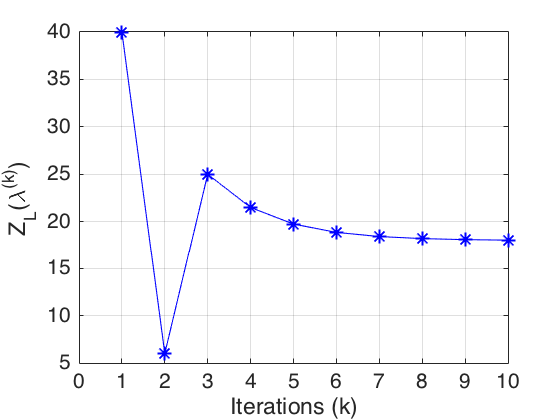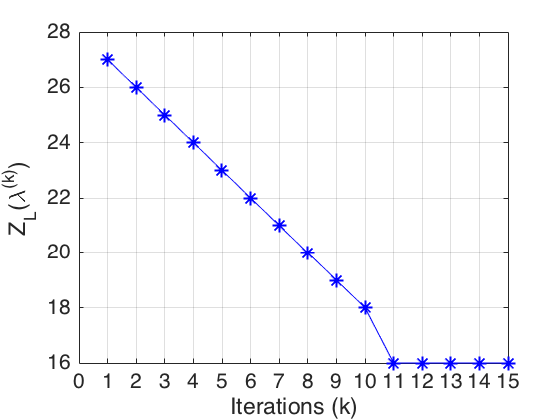$\newcommand{\CF}{\mathcal{F}} \newcommand{\CI}{\mathcal{I}} \newcommand{\CJ}{\mathcal{J}} \newcommand{\CL}{\mathcal{L}} \newcommand{\RR}{\mathbb{R}} \newcommand{\NN}{\mathbb{N}} \newcommand{\ZZ}{\mathbb{Z}} \newcommand{\ra}{\rightarrow} \newcommand{\la}{\leftarrow} \newcommand{\inv}{^{-1}} \newcommand{\maximize}{\mbox{maximize }} \newcommand{\minimize}{\mbox{minimize }} \newcommand{\subto}{\mbox{subject to }} \newcommand{\tr}{^{\intercal}}$

# Lagrangian Relaxation¶

Our general optimization problem in this lecture is in the maximization form:

$$\begin{array}{ll} \maximize & f(x) \\ \subto & x \in \CF, \end{array}$$

where

$$\CF = \{x \in \RR^n : g_i(x) \leq 0, i=1, \dots, m\}$$

with $f:\RR^n \ra \RR$ and $g_i:\RR^n \ra \RR$ for $i=1, \dots, m$. The famous mathematician Lagrange came up with a neat idea in 1785. In a nutshell, Lagrane suggested to remove the difficult constraints from the problem and add them to the objective function with some multipliers to obtain a relaxed problem. Using this idea, we can devise a mechanism to solve a series of simpler problems to obtain better and better approximations. Later, this approach evolved into, what is now known as, the Lagrangian relaxation (Nocedal and Wright, 2006).

Let $\lambda_1, \dots, \lambda_m$ be the Lagrange multipliers associated with the $m$ constraints in our general problem. Then, the Lagrangian function becomes

$$\CL(x, \lambda) = f(x) - \sum_{i=1}^m \lambda_i g_i(x),$$

where $\lambda$ is the vector of $m$ Lagrange multipliers. This leads us to the Lagrangian dual objective function

$$Z_L(\lambda) = \sup_{x \in \RR^n} \CL(x, \lambda).$$

Note for any $\bar{x} \in \CF$ and $\lambda \geq 0$ that the Lagrangian problem satisfies

$$Z_L(\lambda) = \sup_{x \in \RR^n} \{f(x) - \sum_{i=1}^m \lambda_i g_i(x)\} \geq f(\bar{x}) - \sum_{i=1}^m \lambda_i g_i(\bar{x}) \geq f(\bar{x}).$$

If we further define the Lagrangian dual problem

$$\begin{array}{lll} \minimize & Z_L(\lambda) & \\ \subto & \lambda_i \geq 0, & i=1, \cdots, m, \end{array}$$

then we immediately have our weak duality

$$\min\{Z_L(\lambda) : \lambda_i \geq 0, i=1, \cdots, m\} \geq \max\{f(x) : g_i(x) \geq 0, i=1, \dots, m\}.$$

Here is one crucial observation: The Lagrangian function is linear in $\lambda_i$ and hence, the dual objective function is always convex in $\lambda_i$ as it is the supremum of a collection of linear function.

## Applying Lagrangian Relaxation in Integer Programming¶

Like the use of linear programming (LP) relaxation in integer programming (IP), Lagrangian relaxation can also provide a bound for the overall integer problem. As we have discussed in the previous lecture, in a branch and bound application, tight bounds lead to fast pruning of the search tree. For certain problems, we can indeed obtain tighter bounds with the Lagrangian relaxation than those obtained with the LP relaxation. This is, in fact, one of the most important applications of the Lagrangian relaxation.

Consider our example from the previous lecture:

$$\begin{array}{lll} \maximize & x_2 & \\ \subto & -2x_1 + 2x_2 \leq 1, \\ & 2x_1 + 2x_2 \leq 7, \\ & x_1, x_2 \geq 0, \\ & x_1, x_2 \mbox{ integers}. \end{array}$$

The optimal objective function value of the LP relaxation is 2.0 with the optimal solution $(1.5, 2.0)\tr$. Let us now remove the first set of constraints and, with a nonnegative multiplier $\lambda$, create the Lagrangian problem given by

$$\begin{array}{lll} \maximize & x_2 + \lambda(1 + 2x_1 - 2x_2)& \\ \subto & 2x_1 + 2x_2 \leq 7, \\ & x_1, x_2 \geq 0, \\ & x_1, x_2 \mbox{ integers}. \end{array}$$

If we set $\lambda=0.25$, then the optimal objective function value of the Lagrangian problem is 1.75. This is clearly a tighter value than the LP relaxation bound. When we consider the optimal solutions of the Lagrangian problem, we have

$$(3, 0)\tr, ~(2, 1)\tr, ~(0, 3)\tr, ~(1, 2)\tr.$$

Note that the first two solutions are also feasible for the original integer problem, and in fact, the second solution is its optimal.

This elementary example leaves us with three fundamental questions:

1. Which constraints should be removed from the original problem to obtain the Lagrangian dual objective function?
2. What are the good values of the Lagrange multipliers?
3. How can we obtain feasible (incumbent) solutions for the original problem?

First, we need to formalize our discussion. Suppose that we start with the IP problem

$$\begin{array}{rcll} Z_P & = & \maximize & c\tr x \\ & & \subto & Ax \leq b, \\ & & & Dx \leq d, \\ & & & x \geq 0 \mbox{ and integer}, \end{array}$$

where $A$ is $m \times n$ and $D$ is $k \times n$. We remove the first set of constraints and add them to the objective function with the multiplier vector $\lambda \in \RR^m_+$, the resulting Lagrangian dual objective function is given by

$$\begin{array}{rcll} Z_L(\lambda) & = & \maximize & c\tr x + \lambda\tr(b - Ax)\\ & & \subto & Dx \leq d, \\ & & & x \geq 0 \mbox{ and integer}. \end{array}$$

We assume that this problem is relatively easy to solve. Of course, for the tightest bound, we want to solve the Lagrangian dual problem

$$Z_L = \min\{ Z_L(\lambda) : \lambda \geq 0\}.$$

Recall that Lagrangian objective function is convex in $\lambda$. Moreover, this function is differentiable everywhere except at those points where the Lagrangian problem has multiple optimal solutions. Therefore, we cannot directly work with the gradients but instead make use of the subgradients.

Given $\lambda^{(k)}$ at iteration $k$, we need to calculate the new iterate $\lambda^{(k+1)}$. The subgradient method simply states that the new iterate should be along the direction of the subgradient with a properly selected step length, $\alpha_k$. Formally,

$$\lambda^{(k+1)} = \max\{0, \lambda^{(k)} - \alpha_k(b - Ax^{(k)}) \},$$

where $x^{(k)}$ is the optimal solution of $Z_L(\lambda^{(k)})$.

We can best illustrate the method on an example (Fisher, 1985):

$$\begin{array}{rclll} Z_P & = & \maximize & 16x_1 + 10x_2 + 4x_4 \\ & & \subto & 8x_1 + 2x_2 + x_3 + 4x_4 \leq 10, \\ & & & x_1 + x_2 \leq 1, \\ & & & x_3 + x_4 \leq 1, \\ & & & 0 \leq x \leq 1 \mbox{ and integer}. \end{array}$$

If we remove the first constraint with multiplier $\lambda \geq 0$, then the corresponding Lagrangian dual objective function becomes

$$\begin{array}{rclll} Z_L(\lambda) & = & \maximize & (16-8\lambda)x_1 + (10-2\lambda)x_2 + (0-\lambda)x_3 + (4-4\lambda)x_4 + 10\lambda \\ & & \subto & x_1 + x_2 \leq 1, \\ & & & x_3 + x_4 \leq 1, \\ & & & 0 \leq x \leq 1 \mbox{ and integer}. \end{array}$$

We shall first try the subgradient method with different $\alpha_k$ values. First we will keep it constant, then we will reduce each $\alpha_k$ with a factor of $\alpha_{k-1}$.

Homework 2: Check that the optimal value of $\lambda$ is 1 and the optimal objective function value of the integer problem is 16.

In :
numiter = 10; % Just 10 iterations
Aineq = [1, 1, 0, 0; ...
0, 0, 1, 1];
bineq = [1; 1];
options = cplexoptimset; options.Display = 'off'; ctype = 'IIII';

% Constant alphak
lambdak = 0; % Initial value
alphak = 1.0; % Initial value
lambdavals = zeros(numiter,1);
for iter=1:numiter
c = [8*lambdak - 16; 2*lambdak - 10; lambdak; 4*lambdak - 4];
xk = cplexmilp(c, Aineq, bineq, [], [], [], [], [], zeros(4,1), ones(4,1), ctype, [], options);
b_Ax = 10 - [8, 2, 2, 4]*xk;
lambdak = max(0, lambdak - alphak*b_Ax);
lambdavals(iter) = lambdak;
end
plot(lambdavals, 'ro-'); hold on;

% alphak = alphak/2
lambdak = 0;
alphak = 1.0;
lambdavals = zeros(numiter,1);
for iter=1:numiter
c = [8*lambdak - 16; 2*lambdak - 10; lambdak; 4*lambdak - 4];
xk = cplexmilp(c, Aineq, bineq, [], [], [], [], [], zeros(4,1), ones(4,1), ctype, [], options);
b_Ax = 10 - [8, 2, 2, 4]*xk;
lambdak = max(0, lambdak - alphak*b_Ax);
lambdavals(iter) = lambdak;
alphak = alphak/2.0;
end
plot(lambdavals, 'b*-');

% alphak = alphak/3
lambdak = 0;
alphak = 1.0;
lambdavals = zeros(numiter,1);
for iter=1:numiter
c = [8*lambdak - 16; 2*lambdak - 10; lambdak; 4*lambdak - 4];
xk = cplexmilp(c, Aineq, bineq, [], [], [], [], [], zeros(4,1), ones(4,1), ctype, [], options);
b_Ax = 10 - [8, 2, 2, 4]*xk;
lambdak = max(0, lambdak - alphak*b_Ax);
lambdavals(iter) = lambdak;
alphak = alphak/3.0;
end
plot(lambdavals, 'g+-'); xlabel('Iterations (k)'); ylabel('\lambda^{(k)}');
legend('\alpha_k = 1', '\alpha_k = \alpha_{k-1}/2', '\alpha_k = \alpha_{k-1}/3', ...
'Location','northoutside', 'Orientation', 'horizontal');
ax = gca; ax.XTick = 0:numiter; grid on;This plot shows that the step length values $\alpha_k$ play an important role in convergence. When the step lengths are constant, then the $\lambda^{(k)}$ values oscillate between 0 and 2. If we halve the step length at each iteration, then we converge to the optimal value of $\lambda=1$. On the other hand, if we reduce the step length more aggresively and divide the step length by 3 at each iteration, then $\lambda^{(k)}$ values converge to a nonoptimal value.

In fact, Held et. al (1974) have shown the sufficient conditions for the step lengths to obtain convergence. As $k \rightarrow \infty$, if

$$\alpha_k \rightarrow 0 ~ \mbox{ and } ~ \sum^k_{j=1} \alpha_j \rightarrow \infty,$$

then $Z_L(\alpha_k)$ converges to the optimal value of the Lagrangian dual problem, $Z_L$.

A well-known formula that works in practice is given by

$$\alpha_k = \frac{\beta_k (Z_L(\lambda_k) - Z^*)}{\|b - Ax\|^2},$$

where $\beta_k \in (0, 2]$ and $Z^*$ is the best known feasible solution for the original problem. Usually, $\beta_k$ is set to 2 and then halved, if $Z_L(\lambda_k)$ does not change for several iterations. We can try it.

In :
lambdak = 0;
alphak = 1.0;
Zstar = 0; % Initial value
betak = 2; % Initial value
Zstarvals = zeros(numiter,1);
lambdavals = zeros(numiter,1);
ZLkprev = realmax;
for iter=1:numiter
c = [8*lambdak - 16; 2*lambdak - 10; lambdak; 4*lambdak - 4];
[xk, ZLk] = cplexmilp(c, Aineq, bineq, [], [], [], [], [], zeros(4,1), ones(4,1), ctype, [], options);
ZLk = -ZLk + 10*lambdak;
if (ZLkprev < ZLk)
rediter = rediter + 1;
else
rediter = 1;
end
ZLkprev = ZLk;
b_Ax = 10 - [8, 2, 2, 4]*xk;
lambdak = max(0, lambdak - alphak*b_Ax);
lambdavals(iter) = lambdak;
if (b_Ax >= 0)
ZL = [16, 10, 0, 4]*xk;
if (ZL > Zstar)
Zstar = ZL;
end
end
if (rediter == 3) % Objective does not decrease for 3 consecutive iterations
betak = betak/2.0;
rediter = 1;
end
alphak = (betak*(ZLk - Zstar))/(b_Ax^2);
Zstarvals(iter) = Zstar;
end
subplot(2,1,1);
plot(Zstarvals, 'ro-'); xlabel('Iterations (k)'); ylabel('Z*');
ax = gca; ax.XTick = 0:numiter; grid on;
subplot(2,1,2);
plot(lambdavals, 'ro-'); xlabel('Iterations (k)'); ylabel('\lambda^{(k)}');
ax = gca; ax.XTick = 0:numiter; grid on;## Lagrangian Relaxation vs. LP Relaxation¶

Note that we still solve an IP to evaluate the Lagrangian dual objective function for a given $\lambda$. To avoid facing potentially difficult IP problems, one may consider leaving only those constraints so that the LP relaxation of the Lagrangian dual objective function will return integral solutions. Unfortunately, this straightforward idea does not help because in that case, the Lagrangian relaxation bound ($Z_L$) is the same as the bound that we will obtain by solving the LP relaxation of the original problem ($Z_{LP}$).

Here is why:

$$\begin{array}{rl} Z_L& = \min\{Z_L(\lambda): \lambda \geq 0\} \\ & = \min\{\max\{ c\tr x + \lambda\tr(b - Ax): Dx \leq d, x \geq 0 \mbox{ and integer}\} \\ & \leq \min\{\max\{ c\tr x + \lambda\tr(b - Ax): Dx \leq d, x \geq 0\} \\ \end{array}$$

If we denote the dual variables associated with the constraints $Dx \leq d$ by $\mu \in \RR^k$, then we can take the dual of the inner LP problem and obtain

$$Z_L \leq \min\{\min\{ \lambda\tr b+ \mu\tr d: \lambda\tr A + \mu\tr D \geq c, \lambda \geq 0, \mu\geq 0\} = \max\{c\tr x : Ax \leq b, Dx \leq d \} = Z_{LP},$$

where the last equality follows from taking the LP dual once more.

The derivation above shows that if the Lagrangian objective function does not change after we remove the integrality constraints, then $Z_L = Z_{LP}$. To have a tighter bound with the Lagrangian dual problem ($Z_L < Z_{LP}$), we need to put an effort to deal with the IP problem.

This is indeed the case with our example. Both the Lagrangian relaxation bound and the LP relaxation bound are 18.

In :
lambdak = 0;
alphak = 1.0;
zlkvals = zeros(numiter,1);
for iter=1:numiter
c = [8*lambdak - 16; 2*lambdak - 10; lambdak; 4*lambdak - 4];
[xk, ZLk] = cplexmilp(c, Aineq, bineq, [], [], [], [], [], zeros(4,1), ones(4,1), ctype, [], options);
b_Ax = 10 - [8, 2, 2, 4]*xk;
lambdak = max(0, lambdak - alphak*b_Ax);
zlkvals(iter) = -ZLk + 10*lambdak;
alphak = alphak/2.0;
end
plot(zlkvals, 'b*-'); xlabel('Iterations (k)'); ylabel('Z_L(\lambda^{(k)})');
ax = gca; ax.XTick = 0:numiter; grid on;

%LP relaxation of the original problem
Aineqlp = [8, 2, 2, 4; ...
1, 1, 0, 0; ...
0, 0, 1, 1];
bineqlp = [10; 1; 1]; clp = [-16; -10; 0; -4];
[x, fval] = cplexlp(clp, Aineqlp, bineqlp, [], [], zeros(4,1), ones(4,1), [], options);Well, this is discouraging. All our efforts to use the Lagrangian relaxation seems worthless. This occured because our subproblems to evaluate the Lagrangiad dual objective function were too easy to solve. How about an alternative way to apply the Lagrangian relaxation. This time, we will remove the second and the third constraints by using two Lagrange multipliers $\lambda \geq 0$ and $\gamma \geq 0$. Then, the resulting Lagrangian dual objective function becomes

$$\begin{array}{rclll} Z_L(\lambda, \gamma) & = & \maximize & (16-\lambda)x_1 + (10-\lambda)x_2 + (0-\gamma)x_3 + (4-\gamma)x_4 + \lambda + \gamma \\ & & \subto & 8x_1 + 2x_2 + x_3 + 4x_4\leq 10, \\ & & & 0 \leq x \leq 1 \mbox{ and integer}. \end{array}$$

Note that this problem is different than our previous relaxation and its LP relaxation does not necessarily return an integer solution. In fact, this is the well-known knapsack problem. Although the most general knapsack problem is very difficult to solve, for moderately large problems, it can be solved efficiently using dynamic programming. To keep our discussion simple, we shall solve the knapsack problem with an IP solver here.

In :
numiter = 15;
lambdak = 0; gammak = 0; alphak = 1.0;
zlkvals = zeros(numiter,1);
Aineq = [8, 2, 1, 4]; bineq = 10;
for iter=1:numiter
c = [lambdak - 16; lambdak - 10; gammak; gammak - 4];
[xk, ZLk] = cplexmilp(c, Aineq, bineq, [], [], [], [], [], zeros(4,1), ones(4,1), ctype, [], options);
b_Ax = 1 - [1, 1, 0, 0]*xk;
lambdak = max(0, lambdak - alphak*b_Ax);
b_Ax = 1 - [0, 0, 1, 1]*xk;
gammak = max(0, gammak - alphak*b_Ax);
zlkvals(iter) = -ZLk + lambdak + gammak;
end
plot(zlkvals, 'b*-'); xlabel('Iterations (k)'); ylabel('Z_L(\lambda^{(k)})');
ax = gca; ax.XTick = 0:numiter; grid on;
fprintf('The last Lagrangian solution = '); disp(xk');The last Lagrangian solution =      1     0     0     0


Relaxing the second and third constraints has paid off. We have a much tighter bound value of 16, and the very last Lagrangian solution is the optimal solution of the original problem.

1. Nocedal, J. and S. J. Wright (2006). Numerical Optimization, 2nd Edition, New York:Springer.
2. Fisher, M. (1985). An applications oriented guide to Lagrangian relaxation, Interfaces 15:2, 10-21.
3. Held, M. H., Wolfe, P. and H. D. Crowder (1974). Validation of subgradient optimization, Mathematical Programming, 6:1, 62-88.